This guide shows the way to set up tax charges on your store.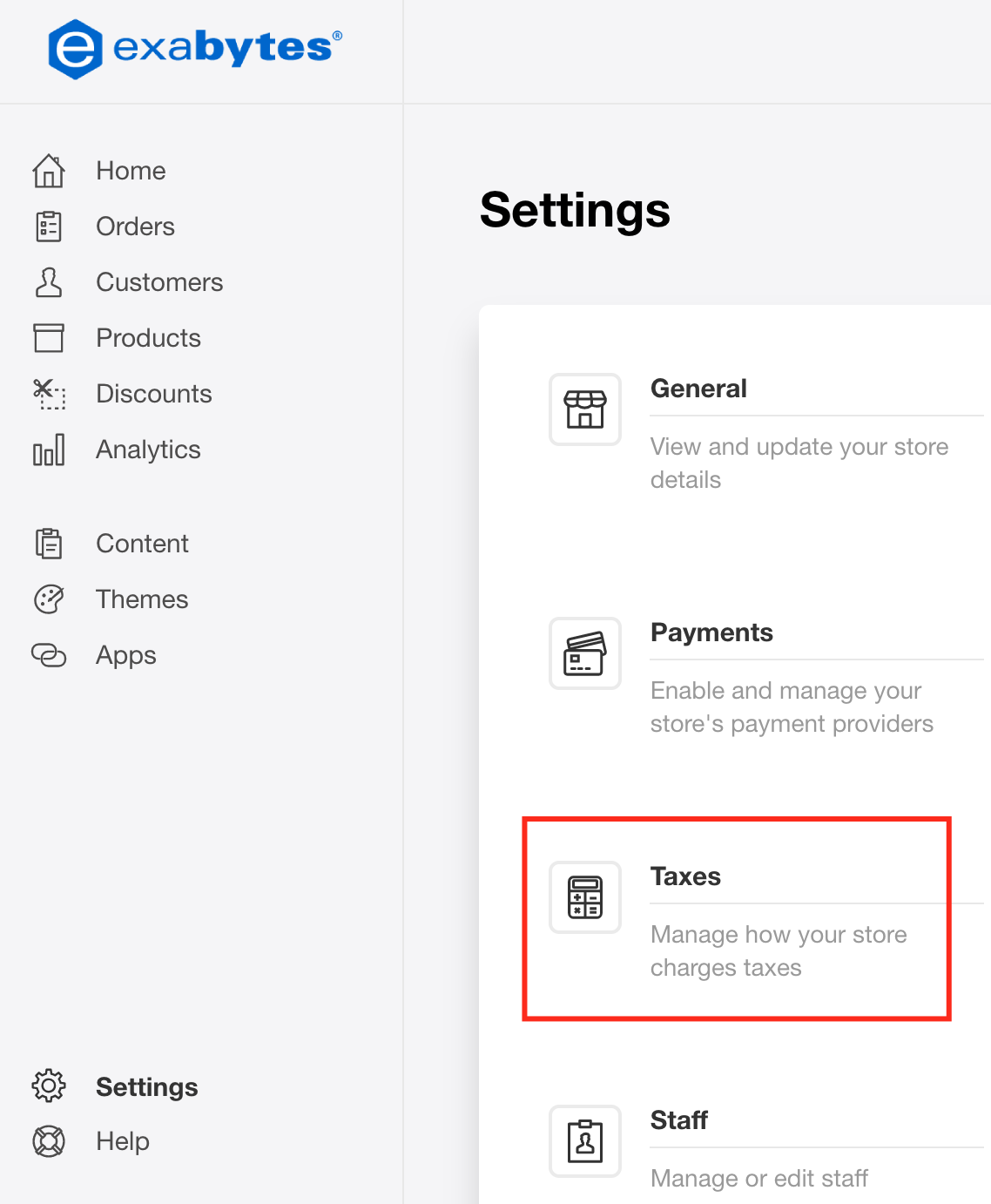2. Select Country > Tax Name > Country Tax3. Manage Sub-regions: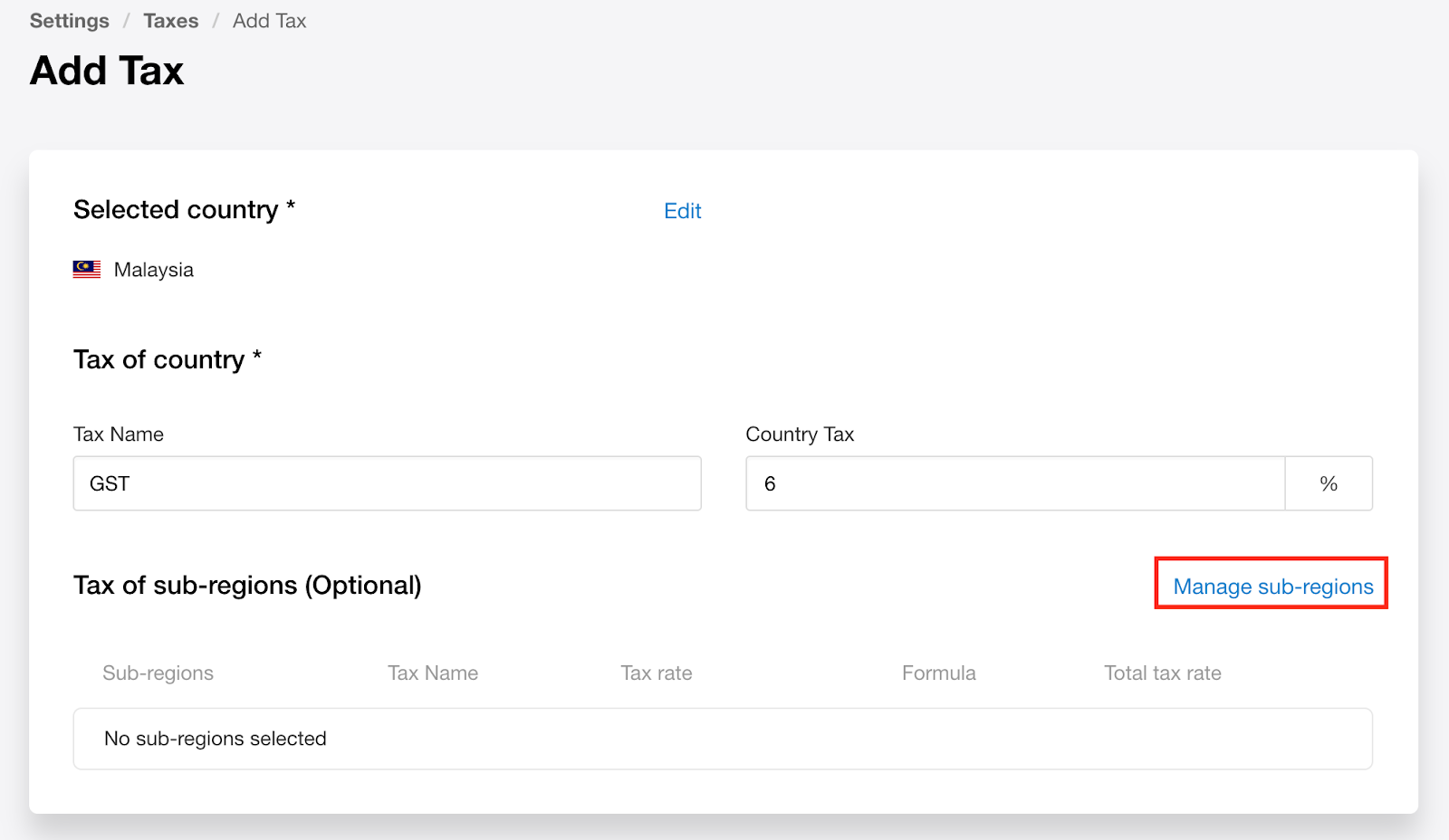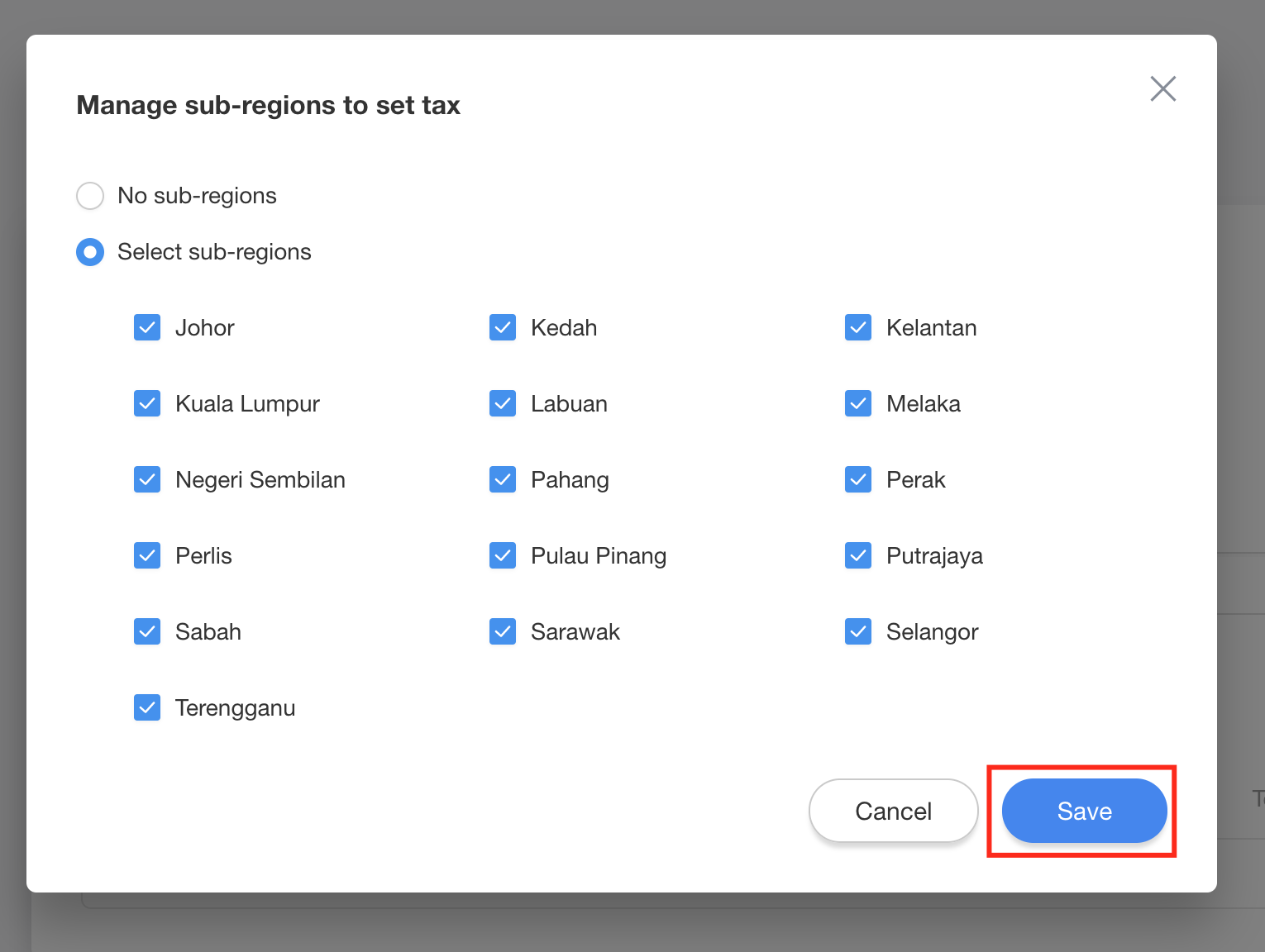4. It would appear a list of sub-regions tax here:Tips:

e.x.

Counrty Tax = 6%,

Tax Rate = 5 %, it will be 5 % + 6 % = 11 %

Calculation as below: Product price = \$ 10.00

\$10.00 * (6 % + 5 %) = \$ 1.10

Total : \$ 10 + \$1.10 = \$ 11.10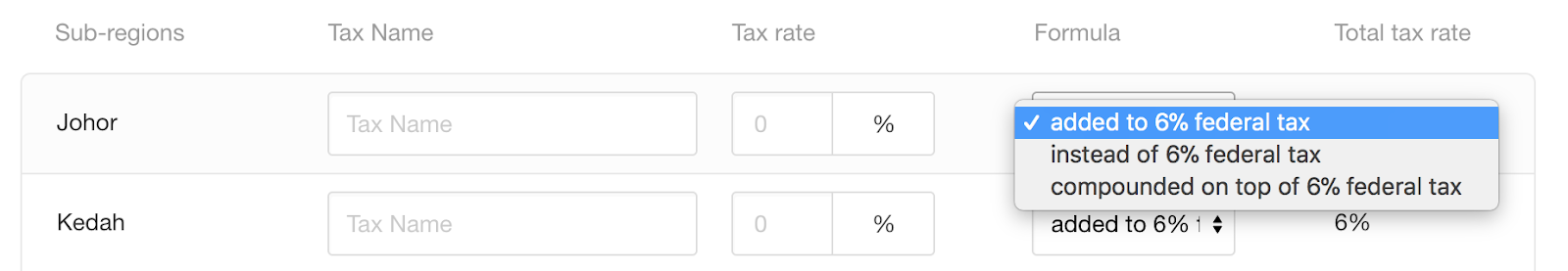Instead of % federal tax - Charge the rate you set for the region instead of the country tax

e.x. Country Tax 6 %,

Tax Rate = 5 %, it will charge 5 % instead of 6 % for the particular region Calculation as below: Product price = \$ 10.00

\$10.00 * 5 % = \$ 0.50

Total : \$ 10 + \$ 0.50 = \$ 10.50Compounded on top of % federal tax - Add % on top of the country tax

e.x.

Country Tax 6 %,

Tax Rate= 5 %, it would charge 5% on top of the 6% tax amount.

Calculation as below: Product price = \$ 10.00

\$10.00 * 6 % = \$ 0.60

Add 5 % on top of 6 % : \$ 0.60 * 5% = \$ 0.03

Total : \$ 10 + \$ 0.60 + \$ 0.03 = \$ 10.63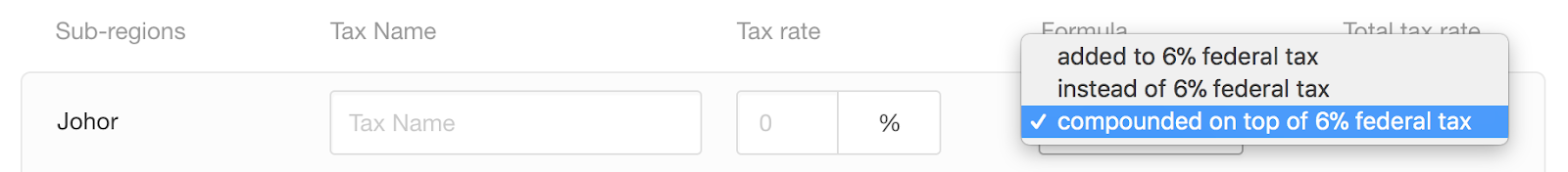5. Choose the way to charge tax: